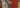Orthorectification is the process of correcting a remote sensing image so that the physical scale across the image is uniform, and the image is correctly aligned with the terrain (or ground) being imaged.

Correctly means that, up to a certain degree, the location of particular features on the image correspond to their real location on the ground.

Performing this process of orthorectifying the image is anything but trivial. There are many factors involved in correctly placing an image on the ground and making sure that the scale is uniform across the image.

Regardless of the platform used for producing the image–aerial or satellite–there will be distortions that are related to the geometry of both the terrain and the sensor, as well as the motion of the platform and the physics of the sensor.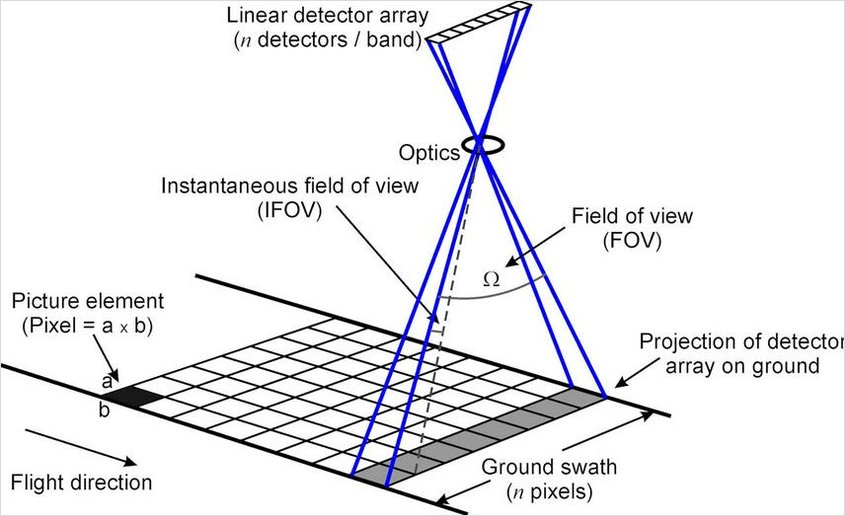Satellite acquiring image at nadir

In an earlier article, we presented an overview of orthorectification. In this article, we're going to delve into the specifics of the process and how to practice it.

These days, performing orthorectification involves optics, geometry, and electronics.

• Optics: imaging is based on optics. Light reflected from the ground reaches the sensor for creating an image. The way the light propagates from the ground to the sensor and the interactions with the medium in between is taken into account.

• Geometry: the sensor has a certain geometry that impacts how the image is formed, and the terrain on the ground has a relief that impacts it also.

• Electronics: currently sensors are usually based on Charged Coupled Devices (CCD) that translate the incoming radiation into an electric charge. How this charge is handled to create the image impacts how accurately the image represents the ground.

The sensor operator takes all these factors into account to give a model of the image formation. This model defines how each point on the ground maps to a pixel within the image.

This mapping can be based on a physical or on a function approximation of this model, when ground coordinates are given as a function of the image pixel coordinates (row, column) without accounting for physical considerations.

## Physical model based orthorectification

In physical model based orthorectification, the sensor operator provides a model of image formation such that the position and velocity of the sensor, the attitude of the platform, and the geometry and physics of the sensor are taken into consideration explicitly. The orbit details and attitude are taken directly and the sensor's physics and geometry appear implicitly in the given model.

Measurements made on the ground before launch are used to properly calibrate the sensor and thus provide an accurate model of the physics involved in the image formation once light hits the sensor.

With the internals of the sensor out of the way, we focus on understanding how a ray of light reflected from a point on the ground reaches the sensor. To determine this, we need:

• Geometrical optics: to determine where and how the image is formed, taking a ray of light from the ground to the sensor.
• Atmospheric radiation: to determine the effects of the gaseous elements in the atmosphere on the path traveled by a ray of light from the ground to the sensor.
• Position, velocity, and attitude of the platform: the ephemeris and the attitude determine the plane of image formation.

Physical model based orthorectification involves disclosing details about the sensor operation, namely the operation of its electronics and how the image is formed in the CCD. This disclosure is something that sensor operators often avoid to retain competitive remote sensing market advantages. In a nutshell, it is treated as a trade secret.

Therefore only legacy sensors have publicly available physical sensor models, e.g., SPOT5. Usually, currently operated sensors do not have publicly available physical models for orthorectification.

## Rational Function Model (RFM)

Rational Function Models (RFM) are a way to approximate a given function based on empirical data. They consist of a rational function, i.e., a ratio of two polynomials, where the coefficients of the polynomials in the numerator and denominator are calculated based on a numerical approximation method to minimize a given error measure.

In the case of orthorectification, this model consists of a pair of rational functions that give a latitude, longitude, and elevation, which return a pixel's coordinates, row and column, that correspond with said functions.

This is called the direct model. The inverse or backward model gives a ground point coordinate (longitude, latitude, elevation) which returns the pixel details (row, column) it corresponds to in the image.

For orthorectifying using the RFM, we only need the inverse model, since we know the region of interest on the ground and we cover the image with a grid where we determine which pixels it corresponds to. To accurately do this a Digital Elevation Model (DEM) is usually required. This requirement can be discarded if the region being imaged has very little relief and an average elevation value is enough. Otherwise, distortions may affect the accuracy of the image positioning.

To better understand how RFM based orthorectification works is instructive to understand how these models are derived. They can be derived based on a physical model or based on measured points on the ground: Ground Control Points (GCPs).

The inverse form of the RFM for normalized image coordinates $r_n, c_n$ (row, column) and normalized ground coordinates $X_n, Y_n, Z_n$ (latitude, longitude, elevation) is: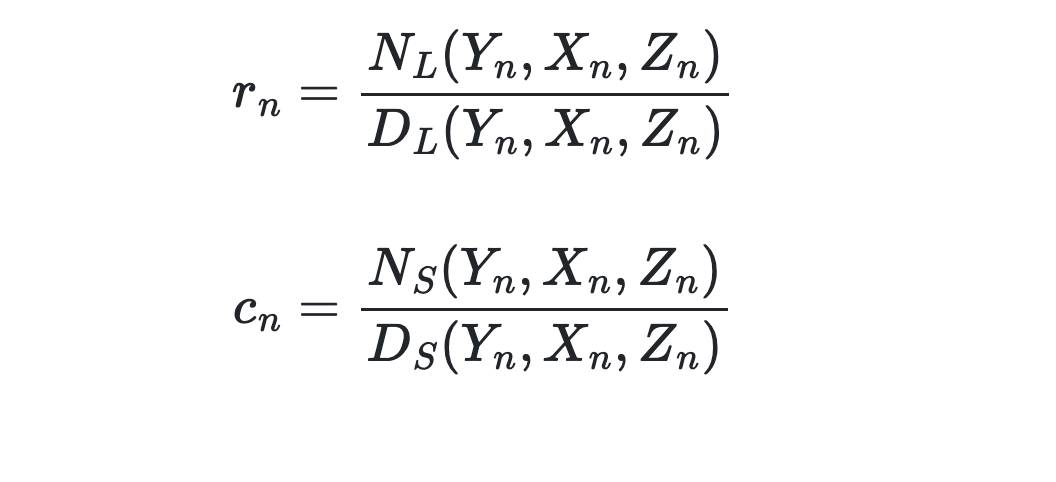with: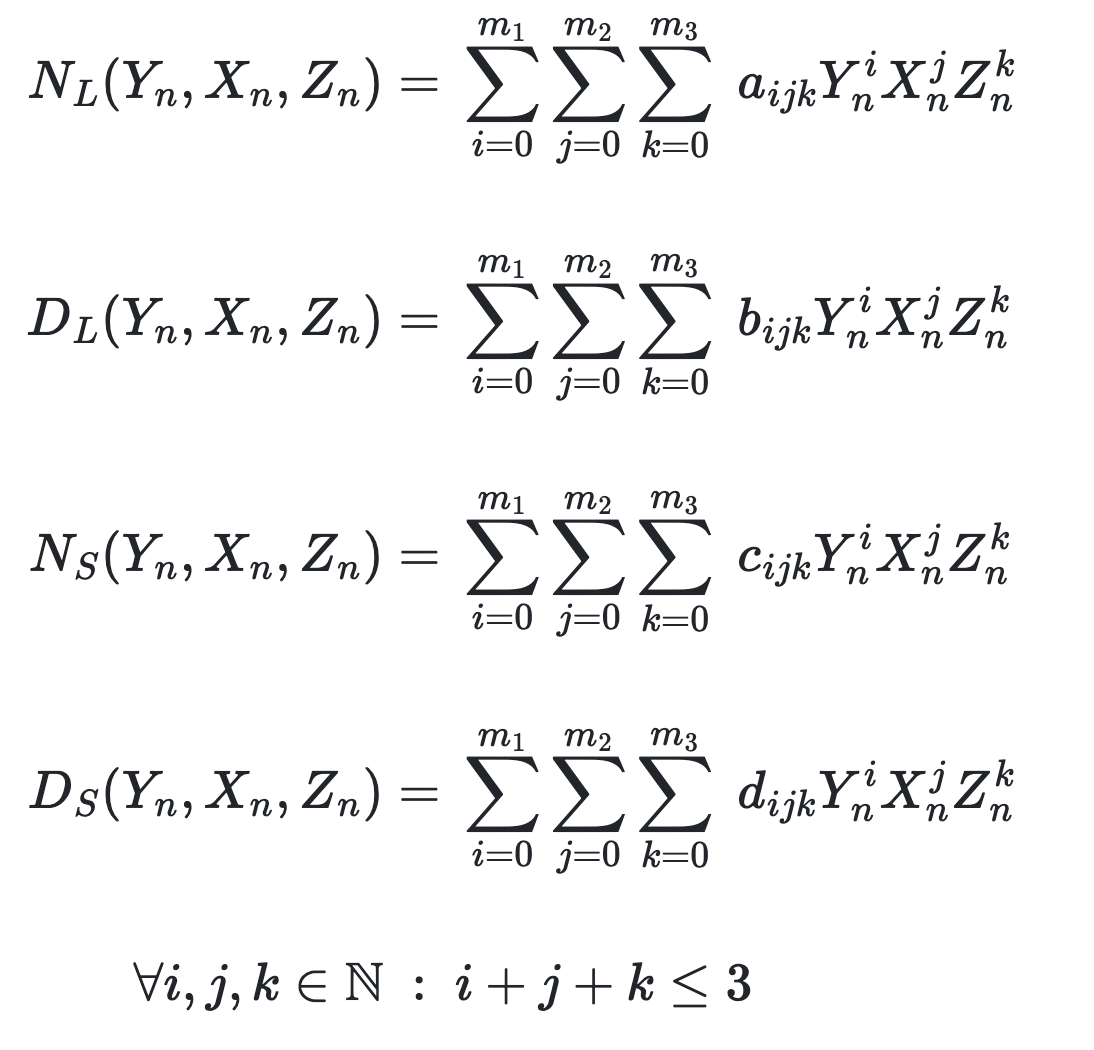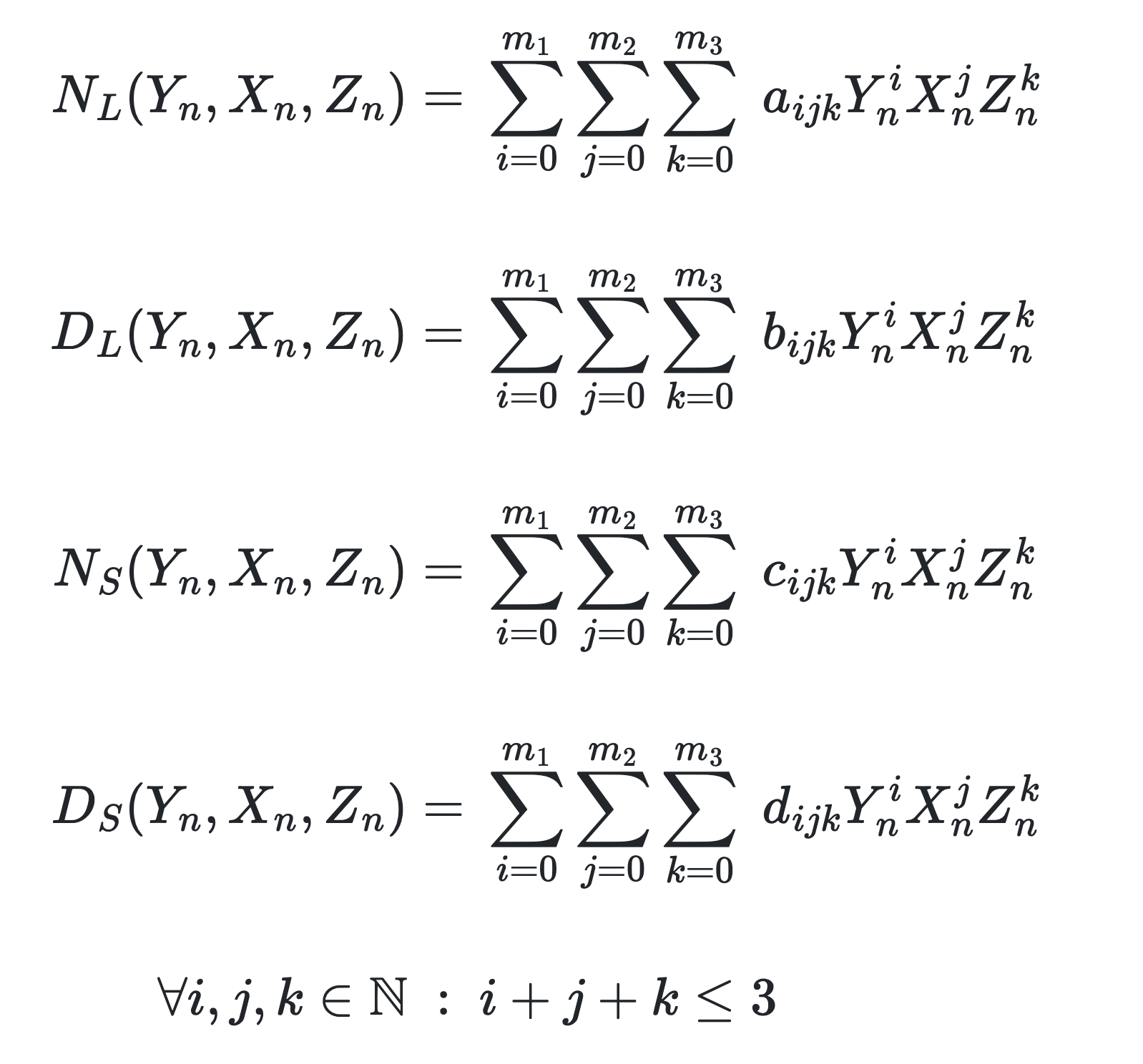and where: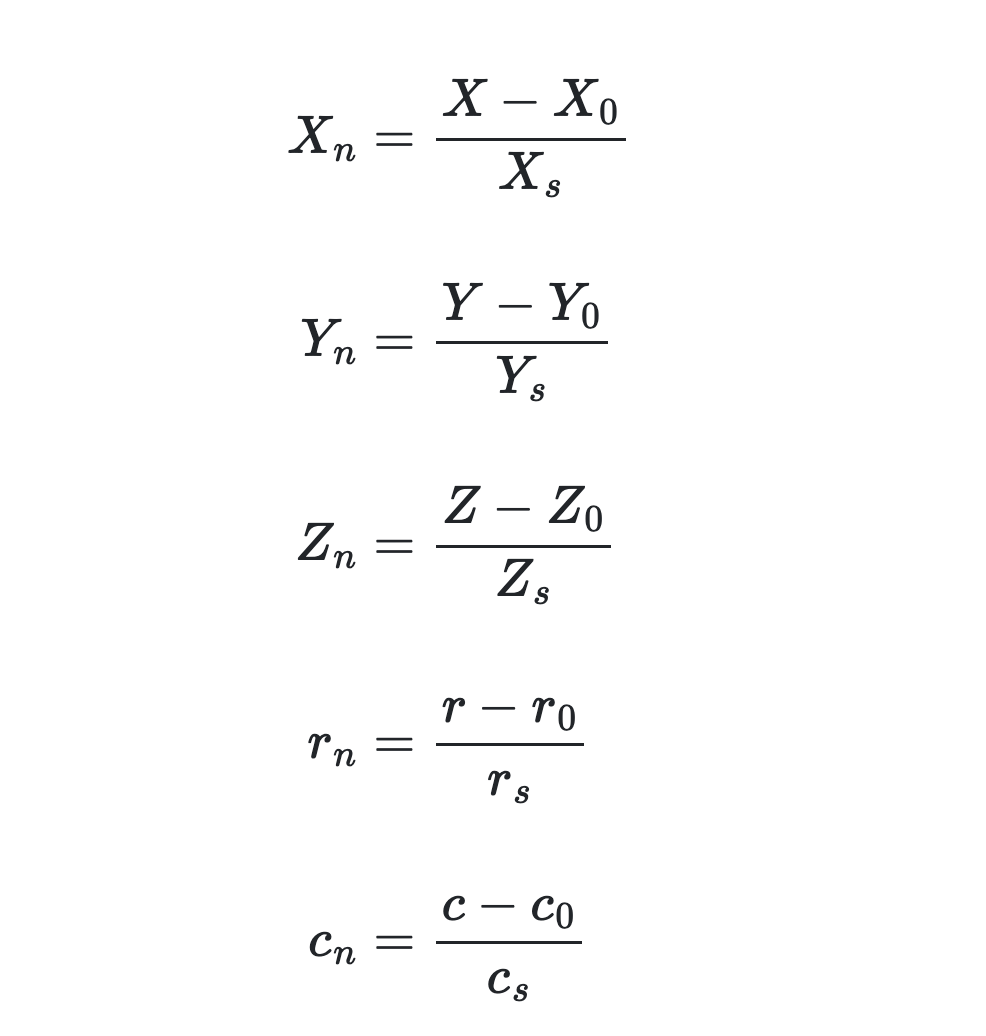$X_s, Y_s, Z_s$ are ground coordinates scale factors and $X_0, Y_0, Z_0$ are the respective offsets. $r_s, c_s$ are image scale factors and $r_0, c_0$ are the respective offsets.

The normalized ground and image coordinates take values in $[-1, 1]$. This is to avoid introducing excessive rounding errors when computing the values of the image coordinates.

The coefficients $a_{ijk}, b_{ijk}, c_{ijk}, d_{ijk}$ are called the Rational Polynomial Coefficients (RPC). The RPC is what is given usually for a remote sensing image, either in a separate metadata file or in file format tags, like in GeoTIFF format. RFM and RPC are both terms used in literature to refer to this type of approximation.

Being a 3rd order polynomial, we can say that [Yuan2009]:

• Distortions caused by the oblique view (optical projection) are modeled in the first-order terms of the RFM.
• Atmospheric effects, lens distortion, and ground curvature are modeled by the second-order terms of the RFM.
• Additional effects like camera vibration and CCD-related anomalies are modeled by the third-order terms of the RFM.

The number of coefficients in each trivariate polynomial is given by $\binom{n+k}{k}$ for $n=3$ (cubic polynomial) and $k=3$ (number of variables). $\binom{6}{3} = 20$. To simplify, the independent terms of the denominators $D_L$ and $D_S$ are set to $1$. Hence, we have $4\times 20 - (1 + 1) = 78$ coefficients in total.

The inverse model is what seems more natural for orthorectification, since it gives us a pixel on the image as a function of the ground coordinates. However, for applications like extraction of digital elevation models (DEMs) from stereo imagery, the direct model is generally used instead.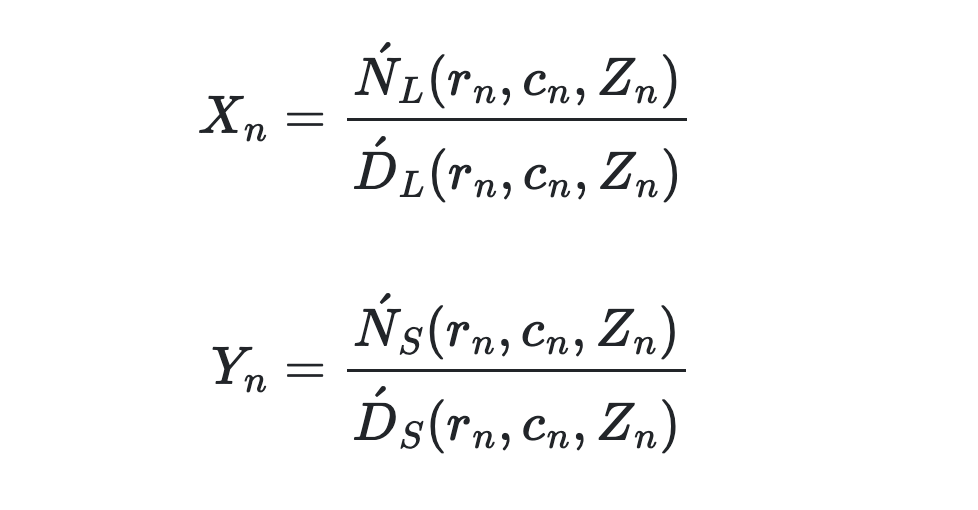The computation of the RPC can be done in one of two ways [TaoHu2001]:

1. Independent of the terrain: a uniform grid on the ground is built using the physical model to get the pixel coordinates given a point. Then the coefficients are computed so that those points are as correct as possible. Using Ground Control Points (GCP) it is possible to iteratively refine the approximation up to a desired level.

2. Dependent of the terrain: a grid of know GCP is superimposed on the image and the coefficients are computed such that the RFM is as correct as possible for those grid control points.

### Terrain-independent RFM

For terrain-independent RFM derivation, we compute the RPC using a point grid obtained from the physical model.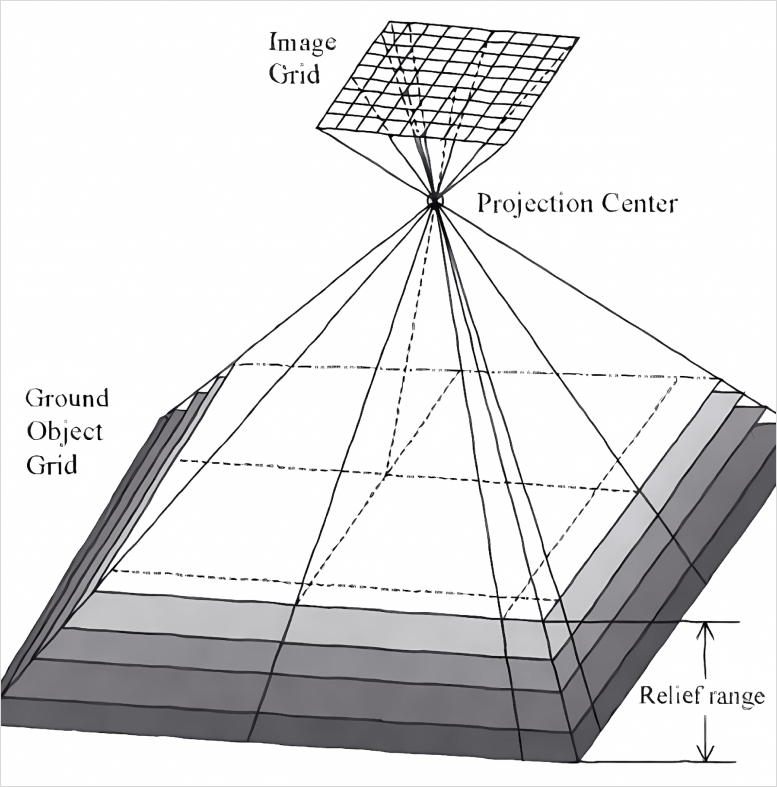Point grid for RPC calculation

There is a horizontal grid and the elevation range is sliced into evenly spaced layers. The number of layers in an elevation model is necessary for accurate ground values of latitude, longitude, and elevation. The elevation is relative to the WGS84 ellipsoid.

A non-linear system of equations involving the RFM must be solved so that the RPC can be estimated. Estimated because using GCP is possible to iterate on the solution so that the residual distance from the GCP to the corresponding RFM compute values is as small as possible.

### Terrain-dependent RFM

Terrain dependent RFM derivation follows a similar process, but now the grid is no longer regular, but instead it follows the positioning of the GCP. See an illustration below of a possible GCP distribution on a given scene on the ground.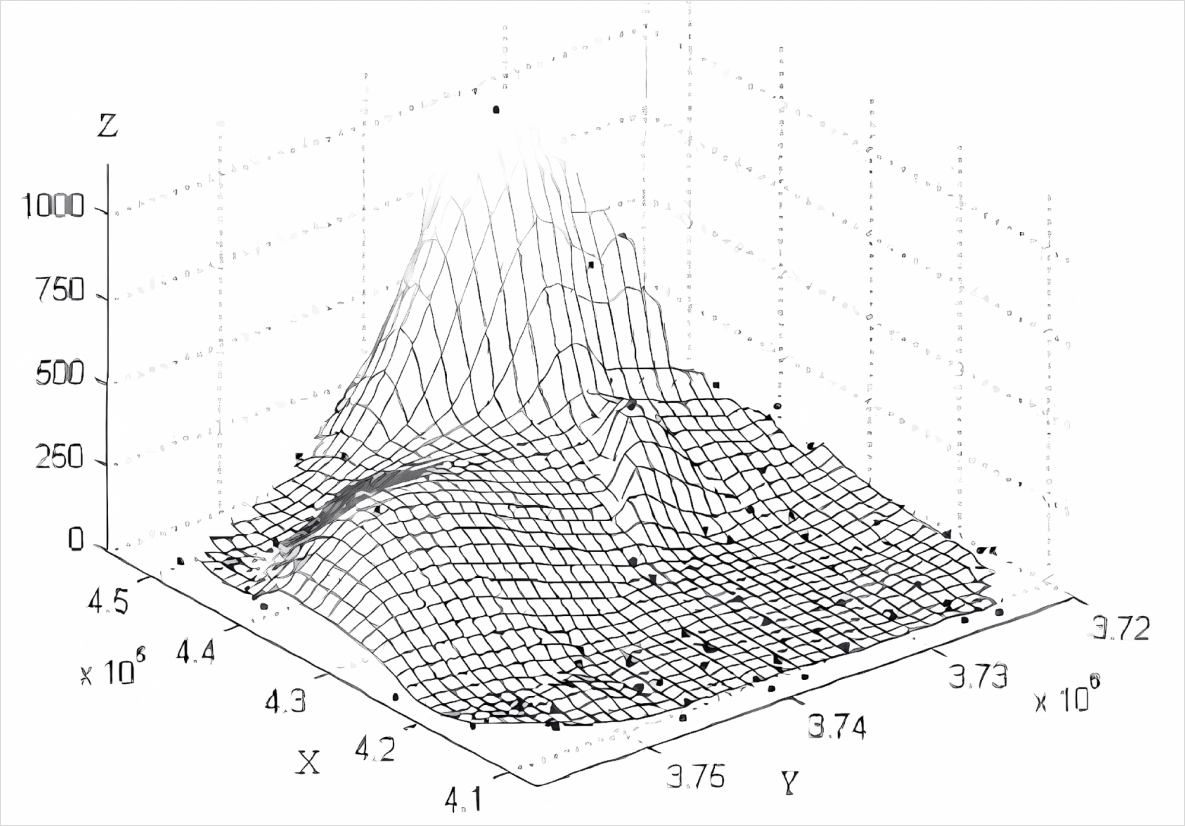Point grid for RPC calculation

## Practical example

Enough theory. Let us orthorectify an image. We are going to use two images from the Airbus sample optical imagery.

to control the quality of the orthrectification we are doing.

the image to be orthorectified.

We also need a DEM in order to provide elevation values to the RFM. We are going to use a WorldDEM Neo product. This product measures elevation against the EGM2008 geoid and as stated above the first thing we need to do is project the DEM from EGM2008 to WGS84.

The tool we are going to use for orthorectification is gdalwarp.

You will need to obtain a datum grid, which is given in the file egm08_25.gtx in a Jupyter notebook.


# Export PATH the the Jupyter magic aliases table.
%rehashx

# Reproject the DEM from EGM2008
%gdalwarp -s_srs "+proj=longlat +datum=WGS84 +no_defs +geoidgrids=egm08_25.gtx" \
-t_srs "+proj=longlat +datum=WGS84 +no_def" \
my_dem.tif my_wgs84_dem.tif

# Let us verify that the the reprojected DEM is in WGS84.

%gdalinfo -proj4 -json my_wgs84_dem.tif | %jq '.coordinateSystem.proj4'

"+proj=longlat +datum=WGS84 +no_defs"

Finally we can proceed to the orthorectification.


# Orthorectify the image.

%gdalwarp -t_srs 'epsg:32613'  -wo SAMPLE_GRID=NO -et 0 -rpc  \
-srcnodata 0 -dstnodata 'None' -to RPC_DEM="my_wgs84_dem.tif" input_primary.tif output_ortho.tif

Let us see how our orthorectified product compares with the Pléiades ortho product. Below is an animation of a detail of the image as given by both the orthorectified image and the data provider orthorectified product.
Differences in detail between orthorectified and ortho product

We can see that the orthorectified image exhibits distortions relative to the ortho product, i.e., the image rectified by the data provider — that uses a physical model and not an RFM —. This might result not only from the approximative nature of the RFM, but also from things as rounding errors in the calculation. It is hard at this stage to pinpoint the exact cause for the degradation. Remember that the horizontal location accuracy is usualluy given within a range of 6.5 m: 6.5 m CE90. Meaning that 90% of the time a point in the image will fall within a 6.5 m radius of a given ground control point, at nadir. More obvious is the deformation of straight lines, e.g., the sides of the road, observed in the orthorectified image compared with the ortho product.

This result seems to confirm that orthorectifying an image is hardly simple, there are many things to consider when doing it.

## Caveats and pitfalls when orthorectifying

There are certain things to keep in mind when doing orthorectification:

• Usually the data provider has a better way of doing orthorectification than we do. Because quite likely it uses a physical model instead of the RFM or other analytical model that approximates the physical model.

• The DEM that is used must not be too coarse or too fine. I.e., if horizontally the DEM grid is not aligned with the original grid then we can be introducing significant errors in the results that the RFM produces, on the other hand, if there are too many points on the DEM horizontal grid, then we might be somewhat outside of the ideal situation for the RFM.

• If you have either a more accurate DEM then is worth trying to perform the orthorectification yourself. Bearing in mind the above caveat that the horizontal resolution of the DEM needs to more or less match the grid.

• If you have at least 3 more precise GCP then you can perform an affine transform of the RFM so that it better aligns with your GCP [Yuan2009].

• If you ordered a stereo ou tri-stereo product for DEM extraction, it makes sense to create the corresponding ortho products yourself. They should be good enough.

## Conclusion

Orthorectification of remote sensing imagery is anything but simple. It requires a thorough understanding of its foundations and of the pitfalls in the way. Most remote sensing analysts and developers are better off trusting the data provider with the orthorectification, rather then doing it themselves. However, as explained in the previous section, there are situations where that might be justified.

Tao, Chao, and Yong Hu. 2001. “A Comprehensive Study of the Rational Function Model for Photogrammetric Processing.” Photogrammetric Engineering and Remote Sensing 67 (December): 1347–57.

Yuan, Xiuxiao. 2009. “Geometric Processing Models for Remotely Sensed Imagery and Their Accuracy Assessment.” In Geospatial Technology for Earth Observation, edited by Deren Li, Jie Shan, and Jianya Gong, 105–39. Boston, MA: Springer US. https://doi.org/10.1007/978-1-4419-0050-0_5.